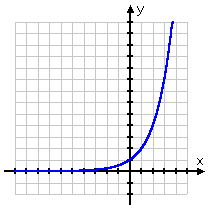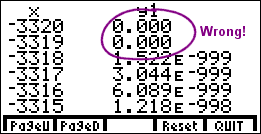Search

Graphing Exponential Functions: Intro (page 1 of 4)

Sections: Introductory concepts, Step-by-step graphing instructions, Worked examplesGraphing exponential functions is similar to the graphing you have done before. However, by the nature of exponential functions, their points tend either to be very close to one fixed value or else to be too large to be conveniently graphed. There will generally be only a few points that are "reasonable" to use for drawing your picture; picking these sensible points will require that you have a good grasp of the general behavior of an exponential, so you can "fill in the gaps", so to speak.

Remember that the basic property of exponentials is that they change by a given proportion over a set interval. For instance, a medical isotope that decays to half the previous amount every twenty minutes and a bacteria culture that triples every day each exhibits exponential behavior, because, in a given set amount of time (twenty minutes and one day, respectively), the quantity has changed by a constant proportion (one-half as much and three times as much, respectively).

 You can see this behavior in any basic exponential function, so we'll use y = 2x as representative of the entire class of functions:On the left-hand side of the x-axis, the graph appears to be on the x-axis. But the x-axis represents y = 0. Can you ever turn "2" into "0" by raising it to a power? Of course not. And a positive "2" cannot turn into a negative number by raising it to a power, so the line, despite its appearance, never goes below the x-axis into negative y-values; the graph of y = 2x is always actually above the x-axis, even if only by a vanishingly-small amount.

So why does it look like it is right on the axis? Remember what negative exponents do: they tell you to flip the base to the other side of the fraction line. So if x = 4, the exponential function above would give us 24, which is 24 = 16 and then flipped underneath to be 1/16, which is fairly small. By nature of exponentials, every time we go back (to the left) by 1 on the x-axis, the line is only half as high above the x-axis as it had been for the previous x-value. That is, while y = 1/16 for x = 4, the line will be only half as high, at y = 1/32, for x = 5. So, while the line never actually touches or crosses the x-axis, it sure gets darned close! This is why, practically speaking, the left-hand side of a basic exponential tends to be drawn right along the axis. If you zoom in close enough on the graph, you will eventually be able to see that the graph is really above the x-axis, but it's close enough to make no difference, at least as far as graphing is concerned.

 If you are using TABLE or some similar feature of your graphing calculator to find plot points for your graph, you should be aware that your calculator will return a y-value of "0" for strongly-negative x-values. Your calculator can carry only so many decimal places, and eventually it just gives up and says "Hey, zero is close enough":But you should not forget that this is just a mark of the limitations of the technology. Like I frequently tell my students: "Student smart; calculator stupid". You need to remember that, no matter what the calculator says, the graph is still above the x-axis; the y-values are still positive, though very, very, very small.   Copyright © Elizabeth Stapel 2002-2011 All Rights Reserved

 Let's look again at the graph of y = 2x:You can see that, on the right-hand side of the x-axis, the graph shoots up through the roof. This is again because of the doubling behavior of the exponential. Once the functions starts visibly growing, it keeps on doubling, so it gets very large, very fast.

You will not generally be plotting many points on the left-hand side of the graph, because the y-values get so close to zero as to make the plot-points indistinguishable from the x-axis. And you will not generally be plotting many point on the right-hand side of the graph, because the y-values get way too big. This is why I've gone on at length (above) about the general shape and behavior of an exponential: You will need this knowledge to help you with the graphing, so make sure you have a fairly good grasp of it.

Top  |  1 | 2 | 3 | 4  |  Return to Index  Next >>

 Cite this article as: Stapel, Elizabeth. "Graphing Exponential Functions: Intro." Purplemath. Available from     https://www.purplemath.com/modules/graphexp.htm. Accessed [Date] [Month] 2016

Study Skills Survey

Tutoring from Purplemath
Find a local math tutor